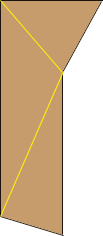Name: Sabrina Who is asking: Other Level of the question: All Question: I am trying to determine how many square feet is in a room that is not square or rectangular. It has basically 5 walls of different lengths, it is acutely considered a hallway in an old house, how can I figure out the footage, the Length times Width requires to much wasted materials...thanks so much... Hi Sabrina, I drew a diagram of a possible configuration of your room.Draw some diagonals, as I have done above, to divide the hallway into triangles, and then the area of the hallway is the sum of the areas of the triangles. The area of each of the triangles can be calculated using 1/2baseheight, if you can easily measure the heights, or you can find the areas of the triangles using Heron's Formula. Penny